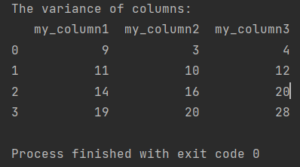Pandas How To Uncategorized How to calculate variance in Pandas

# How to calculate variance in Pandas

Here’s how to calculate variance in Pandas.## How to calculate variance in Pandas

To calculate a variance in Pandas just use a var method which Pandas is offering to you.

```import pandas as pd

my_df = pd.DataFrame({"my_column1": [9, 2, 3, 5],
"my_column2": [3, 7, 6, 4],
"my_column3": [4, 8, 8, 8]})

print(f'The variance of columns:\n{my_df.var()}')

```

The var method calculates the sample variance by default, which is an unbiased estimator of the population variance. The sample variance is calculated as the average of the squared differences between each value in the column and the mean of the column.

```The variance of columns:
my_column1  my_column2  my_column3
0           9           3           4
1          11          10          12
2          14          16          20
3          19          20          28
```

If you want to calculate the population variance, you can set the ddof parameter to 0:

```variance = df['column_name'].var(ddof=0)

```

The ddof parameter stands for degrees of freedom and is used to adjust the denominator of the variance calculation for sample variance (ddof=1) or population variance (ddof=0).

It is important to understand the difference between sample variance and population variance and to set the ddof parameter appropriately when calculating the variance.

For more details see the documentation of var function.

Tags:

## How to use loc with multiindexHow to use loc with multiindex

Using the loc indexer with a multi-indexed pandas DataFrame or Series allows you to subset the data based on one or more levels of the index. Here are the general

## How to pivot tables in Pandas?How to pivot tables in Pandas?

One of the most useful features of Pandas is the ability to pivot tables, which allows you to transform data by grouping, aggregating, and reshaping it.

## How to replace string with another string in PandasHow to replace string with another string in Pandas

To replace a string with another string in a Pandas DataFrame, you can use the str.replace() method. This method allows you to replace a substring within a string with a